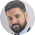# Ratio and Proportions - 6 basic concepts you should know!

Ratio and Proportions is another important topic from banking exam point of view; it can come as an individual question and it can also come as a part of data interpretation.

RPs (short for Ratio and Proportions) are easy enough if we could just get the hang of the basic concept. Today my effort will be to revise the basic premise of RPs and tackle some short cut concepts too.

## Basic Concepts

### 1. Ratio

When two numbers are represented in the form of another; this is done by expressing one number as a fraction of another.

Thus, we have a:b; where a is the  antecedent, and b is the consequent (a little general knowledge doesn't hurt even in math!)

Thus when we write 4:16,
it can be re-written as 2:8 and further simplifying it can be said to be 1:4.

Which actually means, the number ‘4’ is 4 times to get the figure 16.

And all of you know, that 1:4 can also be written as ¼.

### 2. Ratios to percentages:

This 1/4 ratio can be denoted as a percentage too! It’s 25% How?

Well, 1/4 x 100 = 25% [1/4th is also known as one quarter, that is one part out of 4 parts.]

2/4 = 1/2 =50%

3/4 = 75%

and, 4/4 = 100%

### 3. Ratios to Degrees:

Supposing, we have A:B:C:D, being four farmers, who have contributed Rs. 25,000,
Rs. 75,000, Rs. 65,000 and Rs. 35,000 respectively.

Using all their contributions, they have purchased a land, which surprisingly is circular! (C’mon Math need not be boring!)

They decided that they would all receive a part of the circular land based on their contribution; how will they divide the circular land? So one of them who had completed his class 12, suggested they divide the land on a pie chart model!

Total contribution = Rs. 2,00,000 (25000+75000+65000+35000)
Ratio of contribution = 25000:75000:65000:35000
= 25:75:65:35 (always cancel off the 000s first!)
= 5:15:13:7 (this is our last stage, where no more common factors
are possible, where total of the ratios is 40)

We know, if we need A’s share, then A’s ratio = 5/40,
B’s = 15/40,
C’s = 13/40 and D’s = 7/40.
Total area = 360°, then A’s share of the total area/ share of 360° = 360 x 5/40 = 45°
B’s share = 360 x 15/40 = 135°
C’s share = 360 x 13/40 = 117° and D’s = 360x7/40 = 63°

### 4. Comparing two ratios or fractions:

If you are give two fractions, say 5/3 and 7/8, and we need to find out which fraction is greater than the other what do we do?

We can either calculate their percentages, 5/3x100 = 167% and 7/8x100 = 88% (approx values)
So we know, that 5/3 is the greater fraction!

Another method can be to compare the two fractions. And to compare we have to make their denominators equal.

To make their denominators equal, for the first fraction, we multiply 8 to both the numerator and denominator. Therefore the first fraction = 40/24.
The second fraction, by multiplying 3 to make the second fraction = 21/24.

Obviously 40 is greater than 21!

Hence, 40/24 or 5/3 is greater than 21/24 or 7/8!

### 5. Proportions

Proportions is where two ratios are compared and equated.

Where a:b is a ratio and c:d is another ratio, and if they are equal,
then, they can be re-written as a:b :: c:d.    { the ‘::’ sign means ‘equal to’}

Therefore, a:b = c:d

1:2 :: 7:14 , try out the simplifications!

‘a’ and ‘d’ are called extremes as they are in the extreme ends! And ‘b’ and ‘c’ are called means as they are in the middle!

### 6. Properties of proportions

(i) a:b = c:d
can be written as a/b = c/d

which implies, axd = bxc, or, ad = bc
this property helps in solving many questions

(ii) if a:b = b:c, which means this proportions between three numbers is in the form of
‘continued proportions’, as all three numbers are having a connection.

so, a/b=b/c or,
ac=bxb or,
ac=b2

These are the basics of ratio and proportions…and this is the level of banking exam questions.

Practice lots of questions to become and expert in the shortcuts and the way you approach a RPs question.

Till then keep learning and practicing and sharing and giving valuable feedbacks!

Get Quantitative Aptitude Made Easy for the shortcut techniques#### What's trending in BankExamsToday

Smart Prep Kit for Banking Exams by Ramandeep Singh - Download here1.going on another superb article we all aspirants are grateful to you thanks a ton....

1.2.2.great sir keep it up, i think u r going to achieve ur goal soon, ur going on a super speed

3.Sir dvd jo hai math ki hindi mein hai kya

4.sir ibps 2013 clerk quant. ka paper post kar dijiye plzz..
thnku sir...

1.2.thnku sr

5.Thankyou very much sir for your kind help and is there any general awareness material for sbi po exams?

6.sir also provide some other tricks on ration or porpoations...

Thanks for commenting. Follow us on Telegram. Search BankExamsToday